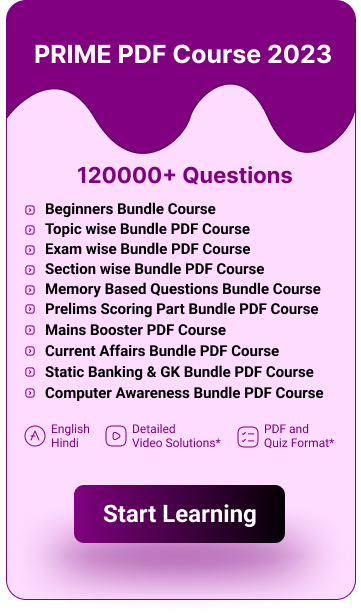# Ratio and Proportion Tricks and Shortcuts For Bank Exams: Download PDF

## Ratio and Proportion Tricks and Shortcuts PDF For Bank Exams

Ratio and proportion tricks pdf is attached to this ratio and proportion tricks for bank exams article. Candidates who are going to appear for future bank exams can make use of this ratio and proportion tricks pdf to solve various types of questions simply with speed and accuracy. Download the Ratio and Proportion Tricks pdf for your future reference.

 Quantitative Aptitude Tricks and Shortcuts PDFs Percentage Average Probability Time Distance Speed Time and Work Permutation and Combination for Bank Exams Boats and Streams Simple interest and compound interest Number system Mixture and alligation Ratio and proportion Pipes and Cisterns Number series Problems on trains Partnership Profit and Loss Quadratic Equation Mensuration Problems on ages

## Ratio and Proportion Tricks and Shortcuts For Bank Exams

Here we come up with the ratio and proportion tricks for bank exams in this ratio and proportion shortcuts for bank exams article. These are the most important ratio and proportion tricks for bank exams which will be very useful for you to solve any type of ratio and proportion questions. So you can learn this most significant ratio and proportion tricks for bank exams here.

• Mean proportion: Mean proportion between x and y is given as √xz

• Third proportion: If p : q = q : s, then s is called as third proportional to p and q.

• Fourth proportion: If u : v = x : y, then y is the fourth proportional of u, v and x.

• Comparison of ratios:-

If ( x : y ) > ( a : b ) = x/y > a/b

• If a/b=x/y, then ay=bx
• If a/b=x/y, then a/x=b/y
• Invertenao: If x/y=z/a, then y/x=a/z
• Componendo: If x/y=z/a, then (x+y)/y=(z+a)/a
• Dividenao: If x/y=z/a, then (x-y)/y=(z-a)/a
• Componendo and Dividendo: If x/y=z/a, then (x+y)/(x-y)=(z+a)/(z-a)
• If x, y, z, and a are four terms and the ratios x : y, y : z, z : a are known, then we can find the ratio x : y : z : a
• If a number a is divided in the ratio x : y,

1) First part:= ax / (x + y)

2) Second part = ay / (x + y)

• Four numbers x, y, z and a are said to be in proportion if x : y = z : a. If on the other hand, x : y = y : z = z : a, then the four numbers are said to be in continued proportion.
• Most important ratio and proportion trick is that you should be proficient in the basic ratio and proportion formula for bank exams. The ratio and proportion formula pdf for bank exams is attached in this ratio and proportion tricks for bank exams post.

We hope that these ratio and prportion tricks and shortcuts for bank exams will useful for your upcoming bank exams and other competitive exams. This ratio and prportion tricks article will help you to increase your ability to solve all the level of ratio and prportion problems. Stay connected on our guidely team to get more details to achieve success in the forthcoming government examinations.

 Quantitative Aptitude Formula PDFs Percentage Average Probability Time Distance Speed Time and Work Permutation and Combination Boats and Streams Simple Interest and Compound Interest Number System Mixture and Alligation Ratio and Proportion Pipes and Cisterns Sequence and Series Problems on Trains Partnership Profit and Loss Mensuration

 Reasoning Tricks and Tips PDFs Seating Arrangement Puzzle Blood Relation Syllogism Coding-Decoding Direction Sense Inequality Input Output

## Ratio and Proportion Tricks and Shortcuts For Bank Exams FAQs

Q. What is the comparison of ratios?

A. Comparison of ratios:-

If ( x : y ) > ( a : b ) = x/y > a/b

Q. What are the important ratio and proportion tricks and shortcuts for bank exams?

A. The important ratio and proportion tricks and shortcuts for bank exams are mentioned in the above article. Kindly go through the above ratio and proportion tricks for bank exams post to know more ratio and proportion tricks for bank exams.

• TAGS

Dec 11 2023

Dec 11 2023

## Easiest Competitive Exams in India 2023: Top 10 Exams To Crack Easily, Bank, SSC, Railway

Dec 11 2023

###### General Awareness Smart Analysis
• Get Weekly 4 set Test
• Each Set consist of 50 Questions
• Compare your progress with Test 1 & 2 & Test 3 & 4
• Deep Analysis in topic wise questions
₹599 ₹99
###### Super Plan
• Bundle PDF Course 2023
• Ultimate Bundle PDF Course 2022
• Grand Bundle PDF Course 2021
• English Bundle PDF Course
• Insurance & Financial Market Awareness Bundle PDF  Course
• Descriptive Papers for Bank & Insurance Exams
• Interview Bundle PDF Course
• General Awareness Smart Analysis
• All Bank Exams Video Course
• All Mock Test Series (Platinum Package)
₹17990 ₹1999
###### Premium PDF Course 12 Months
• Bundle PDF Course 2024
• Prime PDF Course 2023
• Ultimate Bundle PDF Course 2022
₹5999 ₹1199
###### PRIME Bundle PDF Course 2023 (Daily Target PDF)
• Exactly Based on Real Exam Pattern
• Language: English and Hindi
• Included with All New Pattern Questions
• Covered All Major Bank (Pre + Mains Exams)
• Answer key with video solution and Quizzes
• Including Previous Year Memory based Questions
• Prepared by Top Expert Faculties
• Total 1,20,000+ Questions
• 12 Months Validity
₹9999 ₹999